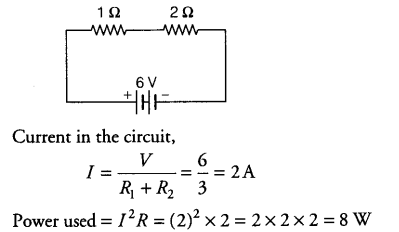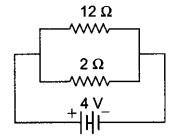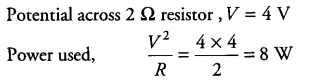# Compare the power used in 2 \$\Omega \$ resistor in each of the following circuits

Compare the power used in 2 \$\Omega \$ resistor in each of the following circuits
(i) a 6 V battery in series with 1 \$\Omega \$ and 2 \$\Omega \$ resistors,
(ii) a 4 V battery in parallel with 12 \$\Omega \$ and 2 \$\Omega \$ resistors.

(i) The circuit shown below has resistance connected in series combination.(ii) The circuit is shown as below:In parallel combination, potential across each resistor is same and equal to the potential applied to the circuit.Power used in both the cases is same.